# Classroom

In a classroom are 30 students, boys are four times more than girls. How many boys are in the class?

x =  24

### Step-by-step explanation:

x+y=30
x = 4y

x+y=30
x = 4•y

x+y = 30
x-4y = 0

x = 24
y = 6

Our linear equations calculator calculates it.Did you find an error or inaccuracy? Feel free to write us. Thank you!Tips to related online calculators
Do you have a linear equation or system of equations and looking for its solution? Or do you have a quadratic equation?

## Related math problems and questions:

• Boys and girls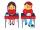In the class are 20 boys and 5-times less girls. How many girls are in the classroom? How many all children are in the class?
• Prospering studentsThere are girls and 30 boys in the class. Boys prospering 28, girls all. How many girls are in the class and how many boys are there, if all the prospering students are 95%?
• The classroom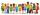In the seventh class the number of boys and girls are at a ratio of 4:5. Boys are 16. How many total students are in the classroom?
• In the classroomThere are 30 boys and a few girls in the class. In the six months, 28 boys and all girls benefited, which was 95% of all pupils. How many pupils are there in the classroom?
• Ski class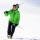Class attend 30 boys and some number of girls. Ski training was attended by 28 boys and all the girls, which was 95% of all students. How many girls attend this class? How many percent is that?
• Classroom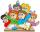In a class are 32 pupils. Boys are 8 less than girls. How many boys and girls are in the classroom?
• PupilsThere are 32 pupils in the classroom, and girls are two-thirds more than boys. a) How many percents are more girls than boys? Round the result to a whole percentage. b) How many boys are in the class? c) Find the ratio of boys and girls in the class. Writ
• Boys and girls 8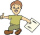In a class of 35 boys and girls that has a ratio of 2:5, 5 boys are absent and 5 boys are present. How many students are present?
• Glasses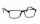There are 36 pupils in the class. Nine girls wear glasses. Boys with glasses are five less than girls without glasses. Boys without glasses are two times more than girls without glasses. How many boys and how many girls?
• GirlsThe boys and girls in the class formed groups without the rest of the fives, two girls and three boys. There are six girls missing to create mixed pairs (1 boy and one girl). How many girls are in the classroom?
• Classroom 4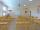In a class of 36 pupils, 2/3 are girls. How many girls and boys are in the class?
• ClassroomThere are eighty more girls in the class than boys. Boys are 40 percent and girls are 60 percent. How many are boys and how many girls?
• A class IV.CIn a class 2/5 were boys. 30 were girls. How many more girls than boys are there?
• ClassroomOf the 26 pupils in the classroom, 12 boys and 14 girls, four representatives are picked to the odds of being: a) all the girls b) three girls and one boy c) there will be at least two boys
• Boys and girlsThere are eight boys and nine girls in the class. There were six children on the trip from this class. What is the probability that left a) only boys b) just two boys
• ClassIn a class are 32 pupils. Of these are 8 boys. What percentage of girls are in the class?
• Competition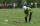15 boys and 10 girls are in the class. On school competition of them is selected 6-member team composed of 4 boys and 2 girls. How many ways can we select students?